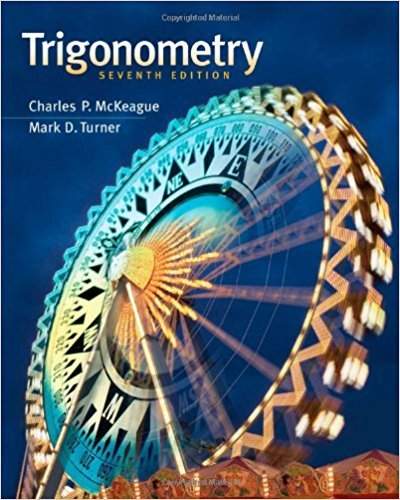×
×

# Solutions for Chapter 8.6: Equations in Polar Coordinates and Their Graphs## Full solutions for Trigonometry | 7th Edition

ISBN: 9781111826857Solutions for Chapter 8.6: Equations in Polar Coordinates and Their Graphs

Solutions for Chapter 8.6
4 5 0 405 Reviews
14
0
##### ISBN: 9781111826857

This expansive textbook survival guide covers the following chapters and their solutions. Trigonometry was written by and is associated to the ISBN: 9781111826857. This textbook survival guide was created for the textbook: Trigonometry, edition: 7. Since 85 problems in chapter 8.6: Equations in Polar Coordinates and Their Graphs have been answered, more than 24949 students have viewed full step-by-step solutions from this chapter. Chapter 8.6: Equations in Polar Coordinates and Their Graphs includes 85 full step-by-step solutions.

Key Math Terms and definitions covered in this textbook
• Augmented matrix [A b].

Ax = b is solvable when b is in the column space of A; then [A b] has the same rank as A. Elimination on [A b] keeps equations correct.

• Cholesky factorization

A = CTC = (L.J]))(L.J]))T for positive definite A.

• Cofactor Cij.

Remove row i and column j; multiply the determinant by (-I)i + j •

• Cross product u xv in R3:

Vector perpendicular to u and v, length Ilullllvlll sin el = area of parallelogram, u x v = "determinant" of [i j k; UI U2 U3; VI V2 V3].

• Eigenvalue A and eigenvector x.

Ax = AX with x#-O so det(A - AI) = o.

• Elimination matrix = Elementary matrix Eij.

The identity matrix with an extra -eij in the i, j entry (i #- j). Then Eij A subtracts eij times row j of A from row i.

• Elimination.

A sequence of row operations that reduces A to an upper triangular U or to the reduced form R = rref(A). Then A = LU with multipliers eO in L, or P A = L U with row exchanges in P, or E A = R with an invertible E.

• Fibonacci numbers

0,1,1,2,3,5, ... satisfy Fn = Fn-l + Fn- 2 = (A7 -A~)I()q -A2). Growth rate Al = (1 + .J5) 12 is the largest eigenvalue of the Fibonacci matrix [ } A].

• Fourier matrix F.

Entries Fjk = e21Cijk/n give orthogonal columns FT F = nI. Then y = Fe is the (inverse) Discrete Fourier Transform Y j = L cke21Cijk/n.

• Gauss-Jordan method.

Invert A by row operations on [A I] to reach [I A-I].

• Incidence matrix of a directed graph.

The m by n edge-node incidence matrix has a row for each edge (node i to node j), with entries -1 and 1 in columns i and j .

• Least squares solution X.

The vector x that minimizes the error lie 112 solves AT Ax = ATb. Then e = b - Ax is orthogonal to all columns of A.

• Linear combination cv + d w or L C jV j.

• Normal equation AT Ax = ATb.

Gives the least squares solution to Ax = b if A has full rank n (independent columns). The equation says that (columns of A)·(b - Ax) = o.

• Normal matrix.

If N NT = NT N, then N has orthonormal (complex) eigenvectors.

• Outer product uv T

= column times row = rank one matrix.

• Pascal matrix

Ps = pascal(n) = the symmetric matrix with binomial entries (i1~;2). Ps = PL Pu all contain Pascal's triangle with det = 1 (see Pascal in the index).

• Similar matrices A and B.

Every B = M-I AM has the same eigenvalues as A.

• Solvable system Ax = b.

The right side b is in the column space of A.

• Toeplitz matrix.

Constant down each diagonal = time-invariant (shift-invariant) filter.

×